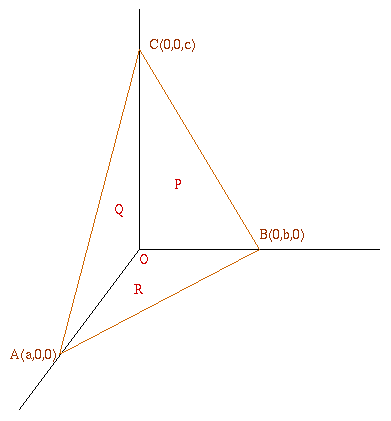#### You may also like### Reach for Polydron

A tetrahedron has two identical equilateral triangles faces, of side length 1 unit. The other two faces are right angled isosceles triangles. Find the exact volume of the tetrahedron.### Tetra Inequalities

Prove that in every tetrahedron there is a vertex such that the three edges meeting there have lengths which could be the sides of a triangle.### The Dodecahedron Explained

What is the shortest distance through the middle of a dodecahedron between the centres of two opposite faces?

# Pythagoras for a Tetrahedron

##### Age 16 to 18Challenge LevelA natural generalisation of Pythagoras' theorem is to consider a right-angled tetrahedron with four faces, three in mutually perpendicular planes and one in the sloping plane. Then ask "what corresponds to the squares of the lengths of the sides?" The answer must be "the squares of the areas of the faces". If these areas are $P$, $Q$ , $R$ and $S$ respectively then prove that: $P^2+ Q^2+ R^2= S^2$
Equivalently: (area $OBC$)$^2 +$(area $OCA$)$^2 +$(area $OAB$)$^2 =$(area $ABC$)$^2$.